# Erik

Three friends Peter, Erik and Milan shared 420 euros in a ratio of 1: 2: 3.
How many euros did Erik get?

e =  140

### Step-by-step explanation: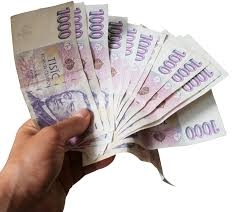Did you find an error or inaccuracy? Feel free to write us. Thank you!Tips to related online calculators
Need help to calculate sum, simplify or multiply fractions? Try our fraction calculator.
Check out our ratio calculator.

## Related math problems and questions:

• Dividing moneyMilan divided 360 euros in the ratio 4: 5, Erik in the ratio 500: 625. How many euros did the individual parts of Milan and how many Erik?
• TogetherThe three friends divided the balls in a ratio of 6: 5: 4. Some two of them got a total of 126 balls. How many balls were there together?
• Moneys in triple ratioMilan, John and Lili have a total 344 euros. Their amounts are in the ratio 1:2:5. Determine how much each of them has?
• Euros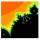Michal, Peter, John and Lenka got together 2,400 euros. They share an amount in ratio 2:6:4:3. How many got each of them?
• Division of moneyCalculate how many euros have Matthew, Miriam, Lucy, Michael, Janka when together have 2,700 euros and the amounts are at a ratio of 1:5:6:7:8.
• EurosPeter, Jane, and Thomas have together € 550. Tomas has 20 euros more than Jane, Peter € 150 less than Thomas. Determine how much has each of them.
• Four friendsFour friends shared the money. Vasek got 1/4 of the total amount. Tonda received 1/3 of the rest of the money, Joe got a half from the second residue and Jirka left 80. How much money get together?
• Three friends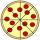Divide 570 euros to three friends so that first will get 50 euros less than the second and third twice more than the first. How many euros will get everyone?
• Tyreek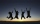Tyreek, Antuan and Alex are given a total of \$3500 to be shared among themselves in the ratio 3:5:12. How much each get money?
• 120 nuts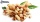Divide 120 nuts in a ratio of 4: 6.
• James 2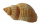James collected 24 seashells on the seashore to be shared with his 2 friends. What fractional part of the seashells will each one get if it is distributed evenly? ​
• Collection of stampsJano, Rado, and Fero have created a collection of stamps in a ratio of 5: 6: 9. Two of them had 429 stamps together. How many stamps did their shared collection have?
• Three friends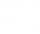Peter is eight times older than Rado, Rado is 3 times younger than Joseph. Together they have 120 years. Determine how many are each of them.
• Word problem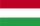348 students were on holiday in Hungary. 133 bought pizza, chips 28 pupils, 25 pupils bought soda, butter 26 pupils, 15 pupils fruits, vegetables 29 students. How much pay for all the food, if every meal cost 5.3 euros?
• Dividing moneyJane and Silvia are to divide 1200 euros in a ratio of 19:11. How many euros does Jane have?
• Borrowing bicycle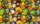Jakub lent a bicycle to friends who wanted to ride it. For a three-hour bike ride, Jakub received 2 chocolates. Yup. If you wanted a bike for 2 hours, you had to give Jakub 12 candies. Peter gave Jakub 1 chocolate and 3 candies. How long can Peter ride Ja
• Jan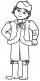Jan is 13 years and Peter 21 years old. For how many years will their ages in the ratio of 7:9?